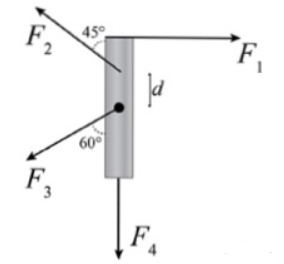# Problem: A uniform thin rod of mass m = 2.6 kg and length L = 1.2 m can rotate about an axle through its center. Four forces are acting on it as shown in the figure. Their magnitudes are F1 = 1.5 N, F2 = 3.5 N, F3 = 11 N, and F4 = 15.5 N, F2 acts as a distance d = 0.28 m from the center of mass.Part A. Calculate the magnitude τ1 of the torque due to force F1 in N•m.Part B. Calculate the magnitude τ2 of the torque due to force F2 in N•m.Part C. Calculate the magnitude τ3 of the torque due to force F3 in N•m.Part D. Calculate the magnitude τ4 of the torque due to force F4 in N•m.Part E. Calculate the angular acceleration a of the thin rod about its center of mass in rad/s2. Let the counter-clockwise direction be positive.

###### FREE Expert Solution

Torque:

$\overline{){\mathbf{\tau }}{\mathbf{=}}{\mathbf{r}}{\mathbf{·}}{\mathbf{F}}{\mathbf{s}}{\mathbf{i}}{\mathbf{n}}{\mathbf{\theta }}}$

Moment of inertia of a rod about its center of mass:

$\overline{){\mathbf{I}}{\mathbf{=}}\frac{\mathbf{1}}{\mathbf{3}}{\mathbf{M}}{{\mathbf{L}}}^{{\mathbf{2}}}}$

Angular acceleration and torque are related by:

$\overline{){{\mathbf{\tau }}}_{\mathbf{n}\mathbf{e}\mathbf{t}}{\mathbf{=}}{\mathbf{I}}{\mathbf{\alpha }}}$

Where I is the moment of inertia.

(A)

F = 1.5 N

r = L/2 = 1.2/2 = 0.6 m

θ = 90°

85% (331 ratings)###### Problem Details

A uniform thin rod of mass m = 2.6 kg and length L = 1.2 m can rotate about an axle through its center. Four forces are acting on it as shown in the figure. Their magnitudes are F1 = 1.5 N, F2 = 3.5 N, F3 = 11 N, and F4 = 15.5 N, F2 acts as a distance d = 0.28 m from the center of mass.Part A. Calculate the magnitude τ1 of the torque due to force F1 in N•m.

Part B. Calculate the magnitude τ2 of the torque due to force F2 in N•m.

Part C. Calculate the magnitude τ3 of the torque due to force F3 in N•m.

Part D. Calculate the magnitude τ4 of the torque due to force F4 in N•m.

Part E. Calculate the angular acceleration a of the thin rod about its center of mass in rad/s2. Let the counter-clockwise direction be positive.